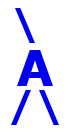## AIRES library reference: fitghfcint.

```c
c     Routine directly callable from C/C++ (it could be necessary to
c     append an underscore, "_", to its name depending on the C/C++
c     compiler used).
c
c
subroutine fitghfcint(bodata0, eodata0, depths, nallch, weights,
+                  ws, minnmax, nminratio, profint,
+                  bodataeff, eodataeff,
+                  nmax, xmax, x0, lambda, sqsum, irc)
c
c     Evaluating the shower maximum parameters (Nmax, Xmax, X0, lambda)
c     via a constrainted four parameter fit of the available shower
c     data to the Gaisser-Hillas function for the profile of charged
c     particles (T. K. Gaisser and A. M. Hillas, in Proc. 15th ICRC
c     (Plovdiv), vol. 8, p. 353 (1977)):
c
c                                   (Xmax - X0)
c                                   -----------
c                      /  X - X0   \  lambda         / Xmax - X \
c        Nch(X) = Nmax | ----------|             exp | -------- |
c                      \ Xmax - X0 /                 \  lambda  /
c
c     Where:
c
c      Nch(X) is the number of charged particles at depth X (in g/cm2).
c      Nmax is the maximum number of particles, Xmax is the depth of the
c      maximum, X0 and lambda are additional varying parameters.
c
c      Nch(X) is zero for X <= X0.
c
c     The constrainted fit is performed imposing that the integral of
c     Nch(X) with respect to X, in the range X0 to infinity is equal
c     to the input parameter "profint" which must be positive.
c
c     Written by: S. J. Sciutto, La Plata 1997, 1998; Fermilab 1999;
c                                La Plata 1999, 2000; Fermilab 2003,
c                                La Plata 2004.
c
c
c     Arguments:
c     =========
c
c     bodata0, eodata0 (input, integer) Positive integer parameters
c                      defining the number of data points to use
c                      in the fit.
c     depths.......... (input, double precision, array(eodata0)) Depths
c                      of the observing levels used in the fit. Only
c                      the range (bodata0:eodata0) is used.
c     nallch.......... (input, double precision, array(eodata0)) Number
c                      of charged particles crossing the different
c                      levels. Only the range (bodata0:eodata0) is
c                      used.
c     weights......... (input, double precision, array(eodata0))
c                      Positive weights to be assigned to each one of
c                      the data points. Only the range
c                      (bodata0:eodata0) is used.
c     ws.............. (input, integer) If ws = 2, the weights are
c                      evaluated internally (proportionally to the
c                      square root of the number of particles).
c                      If ws = 1 they must be provided as input data.
c     minnmax......... (input, double precision) Threshold value for
c                      the maximum number of particles in the input
c                      data set. The fit is not performed if the
c                      maximum number of particles is below this
c                      parameter. If minnmax is negative, it is
c                      taken as zero.
c     nminratio....... (input, double precision) Positive parameter
c                      used to determine the end of the data set.
c                      Must be equal or greater than 5. Once the
c                      maximum of the data set is found. the points
c                      located after this maximum up to the point
c                      where the number of charged particles is less
c                      than the maximum divided nminratio. The
c                      remaining part of the data is not taken into
c                      account in the fit. A similar analysis is
c                      performed with the points located before the
c                      maximum. The recommended value is 100. A very
c                      large value will enforce inclusion of all the
c                      data set.
c     profint......... (input, double precision) Positive number
c                      that gives as input the integral
c
c                                infty
c                                 INT   Nch(X) dX
c                                  X0
c                      whose value is kept fixed during the
c                      constrainted fit.
c     bodataeff,
c     eodataeff....... (output, integer) The actual range of data
c                      points used in the fit.
c     nmax............ (output, double precision) Estimated number of
c                      charged particles at the shower maximum. If no
c                      fit was possible, then the value coming from a
c                      direct estimation from the MC data is returned.
c     xmax............ (output, double precision) Fitted position of
c                      the shower maximum (g/cm2). If no fit was
c                      possible, then the value coming from a direct
c                      estimation from the MC data is returned.
c     x0.............. (output, double precision) Fitted position of
c                      the point where the GH function is zero.
c     lambda.......... (output, double precision) Fitted parameter
c                      lambda.
c     sqsum........... (output, double precision) The resulting
c                      normalized sum of squares:
c
c                                                              2
c                                  1       N   [Nch(i) - GH(i)]
c                      sqsum = ---------- SUM -------------------
c                               N * Nmax  i=1        GH(i)
c
c                      (N is the number of points used in the fit).
c     irc............. (output, integer) Return code. Zero means that
c                      the fit was successfully completed.
c
c
c<--->
```

 These pages are maintained by Sergio J. Sciutto Back to AIRES library index Back to AIRES home